News Group
Dingfeng Capacitor-- Identification of Common Capacitors
Issue Time:2019-01-21

There are two main parameters of capacitor: capacity and rated withstand voltage. The capacity value marked on the capacitor is called nominal capacity. The nominal capacity of the capacitor is only different from the actual capacity, and then divided by the percentage of nominal value is the allowable error.

1. The allowable error of capacity is directly marked on the capacitor.

2. The Roman numbers I, II and III are used to denote (+5%), (+10%) and (+20%) respectively.

3. Use English letters to express the error grade, such as J, K, M and N to express (+5%, +10%, +20%, +30%); D, F and G to express (+0.5%, +1%, +2%); P, S and Z to express (+100%~0%, +50%~20%, +80%~20%) respectively.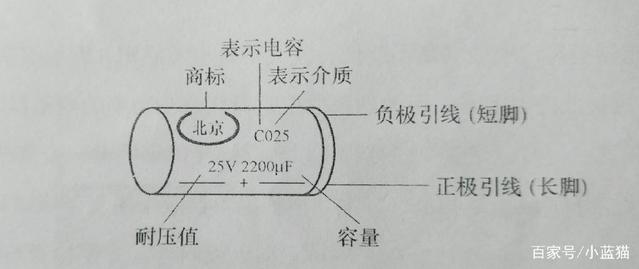1. Direct labeling method. The method of directly marking the main parameters and technical indicators of the product on the surface of the product, such as: 33F + 5%, 32V on the capacitor. If it is zero, the integer "0" is often omitted, such as. 01F for 0.01F.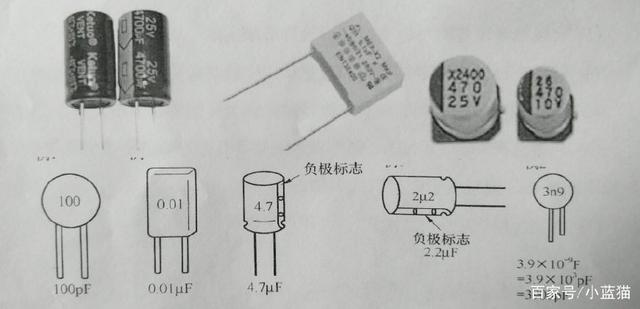2. Character symbol method. The main parameters and technical performance of the logo will be required to regularly combine the logo on the surface of the product with words and integer symbols. When using the character symbol method, the integer part of the capacity is written in front of the capacity unit symbol, and the decimal part is placed behind the unit symbol. For example, the 3.3pF mark is 3p3, the 1000pF mark is 1n, the 6800 mark is 6n8, and the 2.2F mark is 2. (1 farad (F) = 1000 millimeter method (mF) = 1000 000 millimeter method (muF); 1 millimeter method (muF) = 1000 nanometer method (nF) = 1000 000 skin method (pF))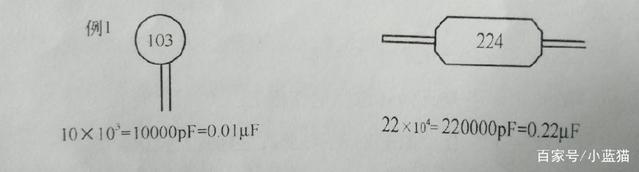3. Digital representation. Number representation is a direct representation of only labeled numbers without labeled units. This method is limited to units F and pF. Generally, the default unit of non-polar capacitor is pF, and the default unit of electrolytic capacitor is F.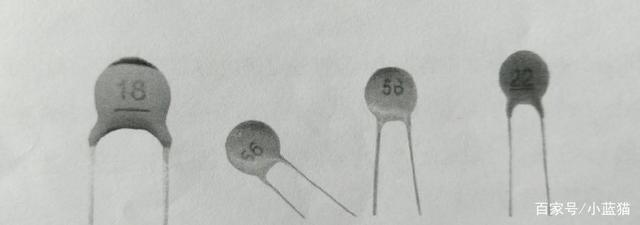4. Digital representation. The commonly used digital labeling method for capacitors with small size generally uses three-digit integers, the first and second digits are valid numbers, and the third digit represents the number of zeros after the valid digits, i.e. multiples, in units of skin method (pF); but when the third digit is 9, it means 10, for example, "243" means capacity of 24000 pF, while "339" means capacity of 33x10 pF (3.3 pF).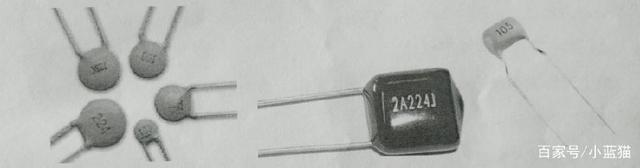5. Color code representation. The colour mark method of capacitor capacity is similar in principle to that of resistors (bits). (The identification method of common resistors has been written before.) The color is applied to one end of the capacitor or arranged from the top to the lead. Color codes generally have only three colors, the first two rings are valid numbers, the third ring is bit rate, capacity unit bit pF.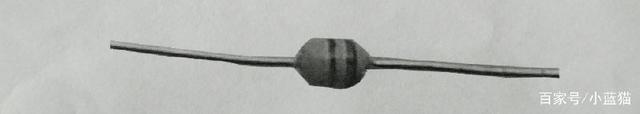6. Hybrid representation of letters and numbers. The mixed alphanumeric representation uses two to four digits and one letter to represent nominal capacity, in which numbers represent valid values and letters represent units of values. Letters sometimes denote units and decimal points.

Voltage withstand value is usually directly labeled on capacitors, but some electrolytic capacitors use color dots at the root of the positive pole to indicate the withstand voltage level, such as 6.3V with brown, 10V with red, 16V with gray. Capacitors are not allowed to exceed this withstand voltage value when they are used. If they exceed this value, the capacitors may be damaged or broken down, or even burst.